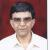true

Learn Python Training from the Best Tutors

•Affordable fees
•1-1 or Group class
•Flexible Timings
•Verified Tutors

Search in

# Writing Simple programs in Python(cont.)Ashish K Sharma
29/11/2021 00

To start writing simple programs in Python, we have to know firstly the SW development process and then we also have to know a bit about the language in which we wish to code,in this case,python, to get started. Since all programs take some kind of input,process it and then produce the results,if we know just how to get data from the user,process it and produce output in a readable form, we would be well on the road to learning how to code. We begin by looking at the notion of a variable.

What is a variable?

Simply stated,a variable is name assigned to a value. For example,

n = 5

here, n is a variable given the value 5.Whenever n appears in an expression, its value,5 will be substituted. Now,let us take a look at some basic mathematical operators in Python.

Some basic Arithmetic Operators.

- = Subtraction

* = Multiplication

/ = Division

** = Exponentiation.

Now,we will look at basic I/O.

Basic I/O

In order to get information from the user, Python uses the input statement,as shown below

This statement,when executed by the Python interpreter,display the string within quotes,prompting the user to enter his name,as shown below:

Here,Rajesh is our response.

Now, to display information to the user, we use the function print. It may be used just to display a message or output the value of a variable or a result of some processing or to display a combination of some strings and variables.

Thus, in Python, input is used to get information from the user and print is used to display information on the screen.

In my earlier lesson, we saw a program in Python which calculates the future value of an investment. Those of you who could not fully understand the program then now will be able to,in light of the elements discussed here(I/O statements,operators etc),comprehend fully the program now. Let us take another example of a simple program in python which calculates the Fahrenheight equivalent of a given celsius temperature. Our program should take a celsius temperature from the user and then print the fahrenheight equivalent. Simple! The algorithm of the program is:

Input the temperature in degrees Celsius (call it celsius)
Calculate fahrenheit as 9/5 celsius + 32
Output fahrenheit.

And the full program in Python:

Note:Take input from the user.

cel = input("What is the Celsius Temperature")

x = 9/5
y = x * int(cel)

fint = y + 32

Note: I have broken the formula of calculating celsius temperature into fahrenheight into

steps for easy calculation. int(cel) is used for converting the value into an int. I have

used it my interpreter,Python 3.3.1 which was not interpreting it correctly without the

int conversion.

Note : Display output.
print("Fahrenheight Temperature = "+str(fint))

So,thus,I hope that users will now be able to start writing simple programs in Python on their own. Of course this is just the beginning! As they progress and learn more details of the language, they will be able to write more sophisticated programs in Python!

Note : Here and in my earlier lesson, I have assumed that students know how to start the IDLE of Python and write and run a program in it. Although the process is quite simple I intend, in the next lesson, to teach students how to create and run a Python Program using the Python IDLE,in case they dont know! Not only we must know the language reading from a book,we must also know how to write a program in it,run it and test it! And with that Students will be able to write simple programs easily enough!

0 Dislike
Follow 1## Other Lessons for You

File Handling in Python - Basic Concept
File (Flat) Handling in Python Types of files in python: 1: Text file: Stores data in the form of characters. Customarily used to store text/string data. 2: Binary file: Stores data in the form of bytes....DBMS - SQL - Any/All
All - Operator SELECT empno, sal FROM emp WHERE sal > ALL (1999, 2999, 3999); Output of Above query is same as below query SELECT empno, sal FROM emp WHERE sal > 1999 AND sal > 2999...Write your first Python program in 10 minutesCode: Gantt Chart: Horizontal bar using matplotlib for tasks with Start Time and End Time
import pandas as pd from datetime import datetimeimport matplotlib.dates as datesimport matplotlib.pyplot as plt def gantt_chart(df_phase): # Now convert them to matplotlib's internal format... ...### Looking for Python Training classes?

Learn from Best Tutors on UrbanPro.

Are you a Tutor or Training Institute?

Join UrbanPro Today to find students near you
X

### Looking for Python Training Classes?

The best tutors for Python Training Classes are on UrbanPro

• Select the best Tutor
• Book & Attend a Free Demo
• Pay and start Learning### Learn Python Training with the Best Tutors

The best Tutors for Python Training Classes are on UrbanProUrbanPro.com is India's largest network of most trusted tutors and institutes. Over 55 lakh students rely on UrbanPro.com, to fulfill their learning requirements across 1,000+ categories. Using UrbanPro.com, parents, and students can compare multiple Tutors and Institutes and choose the one that best suits their requirements. More than 7.5 lakh verified Tutors and Institutes are helping millions of students every day and growing their tutoring business on UrbanPro.com. Whether you are looking for a tutor to learn mathematics, a German language trainer to brush up your German language skills or an institute to upgrade your IT skills, we have got the best selection of Tutors and Training Institutes for you. Read more